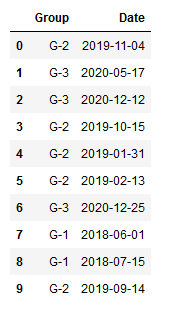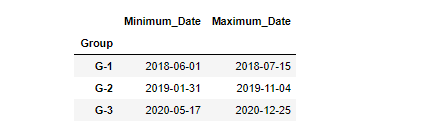# Max and Min date in Pandas GroupBy

• Last Updated : 24 Jan, 2021

Prerequisites: Pandas

Pandas GroupBy is very powerful function. This function is capable of splitting a dataset into various groups for analysis.

Syntax:

`dataframe.groupby([column names])`

Along with groupby function we can use agg() function of pandas library. Agg() function aggregates the data that is being used for finding minimum value, maximum value, mean, sum in dataset.

Syntax:

dataframe.agg(dictionary with keys as column name)

### Approach:

• Import module
• Use GroupBy function on column that you want
• Then use agg() function on Date column.
• Display result

Data frame in Use:Program:

## Python3

 `import` `pandas as pd ` `import` `numpy as np ` ` `  `# Creating Dataframe ` `dataset ``=` `{``'Group'``: [``'G-2'``, ``'G-3'``, ``'G-3'``, ``'G-2'``, ``'G-2'``,  ` `                     ``'G-2'``, ``'G-3'``, ``'G-1'``, ``'G-1'``, ``'G-2'``], ` `            `  `           ``'Date'``: [``'2019-11-04'``, ``'2020-05-17'``, ``'2020-12-12'``,  ` `                    ``'2019-10-15'``, ``'2019-01-31'``, ``'2019-02-13'``, ` `                    ``'2020-12-25'``, ``'2018-06-01'``, ``'2018-07-15'``, ` `                    ``'2019-09-14'``]} ` ` `  `dataset ``=` `pd.DataFrame(dataset, columns``=``[``'Group'``, ``'Date'``]) ` ` `  `# using groupby() function on Group column ` `df ``=` `dataset.groupby([``'Group'``]) ` ` `  `# using agg() function on Date column ` `df2 ``=` `df.agg(Minimum_Date``=``(``'Date'``, np.``min``), Maximum_Date``=``(``'Date'``, np.``max``)) ` ` `  `# Displaying result ` `display(df2) `

Output:My Personal Notes arrow_drop_up
Recommended Articles
Page :